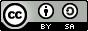# 图像分类¶

## 说明:¶

1.硬件环境要求： 本文可支持在CPU、GPU下运行

2.Docker镜像支持的CUDA/cuDNN版本： 如果使用了Docker运行Book，请注意：这里所提供的默认镜像的GPU环境为 CUDA 8/cuDNN 5，对于NVIDIA Tesla V100等要求CUDA 9的 GPU，使用该镜像可能会运行失败。

3.文档和脚本中代码的一致性问题： 请注意：为使本文更加易读易用，我们拆分、调整了train.py的代码并放入本文。本文中代码与train.py的运行结果一致，可直接运行train.py进行验证。

## 效果展示¶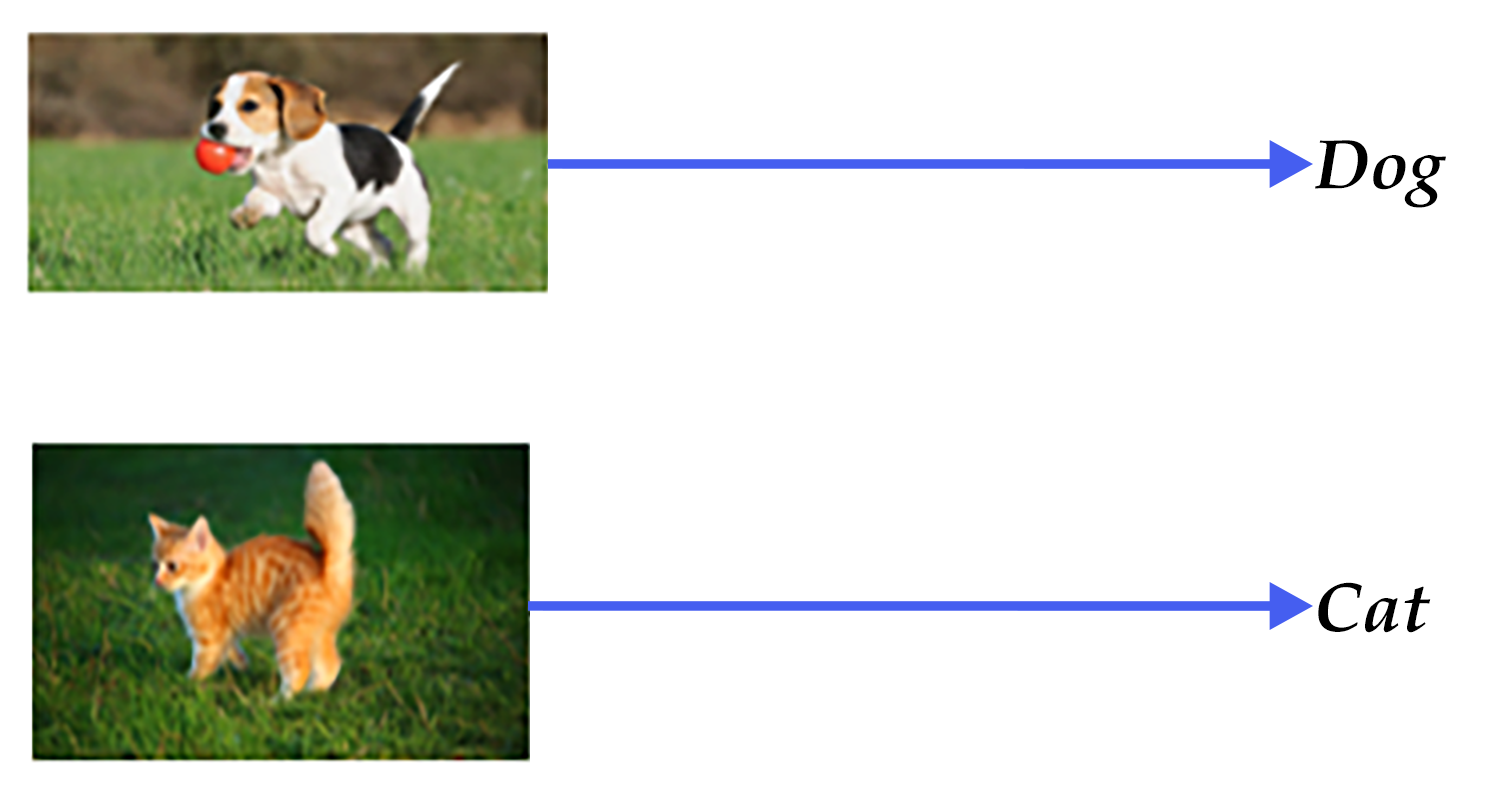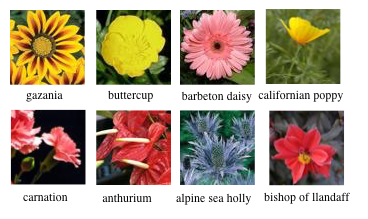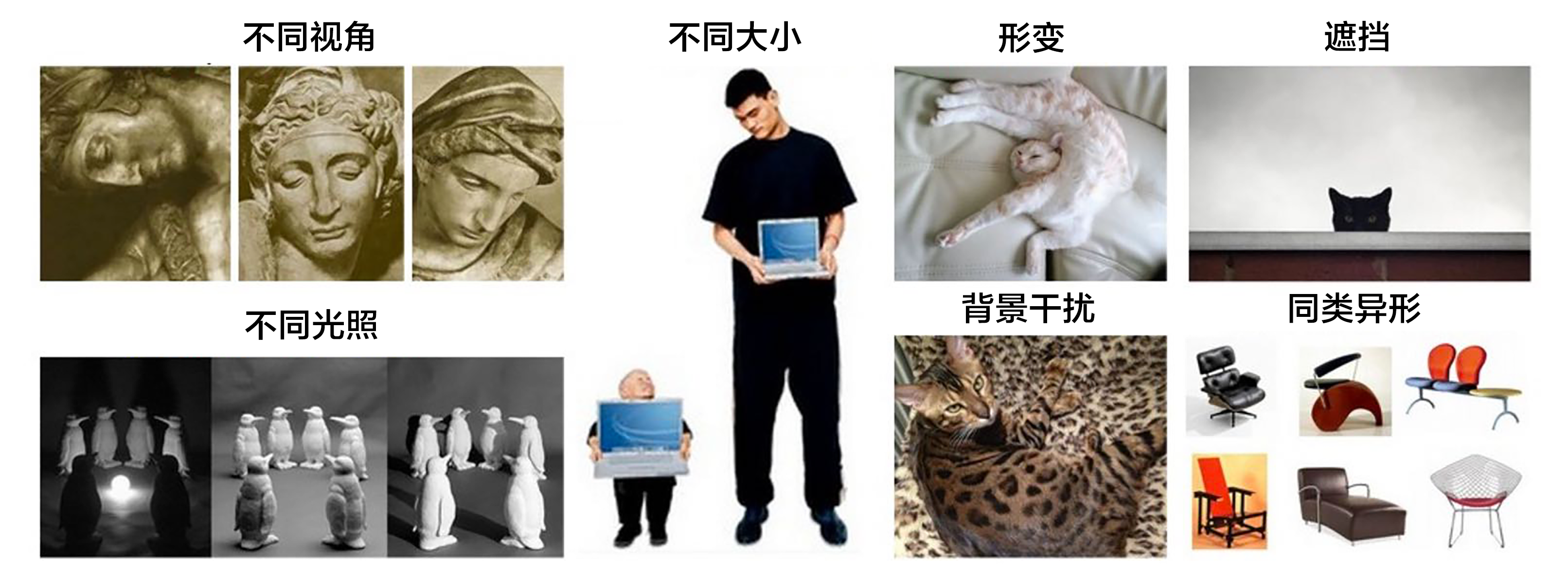## 模型概览¶

1). 底层特征提取: 通常从图像中按照固定步长、尺度提取大量局部特征描述。常用的局部特征包括SIFT(Scale-Invariant Feature Transform, 尺度不变特征转换) 、HOG(Histogram of Oriented Gradient, 方向梯度直方图) 、LBP(Local Bianray Pattern, 局部二值模式)  等，一般也采用多种特征描述，防止丢失过多的有用信息。

2). 特征编码: 底层特征中包含了大量冗余与噪声，为了提高特征表达的鲁棒性，需要使用一种特征变换算法对底层特征进行编码，称作特征编码。常用的特征编码方法包括向量量化编码 、稀疏编码 、局部线性约束编码 、Fisher向量编码  等。

3). 空间特征约束: 特征编码之后一般会经过空间特征约束，也称作特征汇聚。特征汇聚是指在一个空间范围内，对每一维特征取最大值或者平均值，可以获得一定特征不变形的特征表达。金字塔特征匹配是一种常用的特征汇聚方法，这种方法提出将图像均匀分块，在分块内做特征汇聚。

4). 通过分类器分类: 经过前面步骤之后一张图像可以用一个固定维度的向量进行描述，接下来就是经过分类器对图像进行分类。通常使用的分类器包括SVM(Support Vector Machine, 支持向量机)、随机森林等。而使用核方法的SVM是最为广泛的分类器，在传统图像分类任务上性能很好。

Alex Krizhevsky在2012年ILSVRC提出的CNN模型  取得了历史性的突破，效果大幅度超越传统方法，获得了ILSVRC2012冠军，该模型被称作AlexNet。这也是首次将深度学习用于大规模图像分类中。从AlexNet之后，涌现了一系列CNN模型，不断地在ImageNet上刷新成绩，如图4展示。随着模型变得越来越深以及精妙的结构设计，Top-5的错误率也越来越低，降到了3.5%附近。而在同样的ImageNet数据集上，人眼的辨识错误率大概在5.1%，也就是目前的深度学习模型的识别能力已经超过了人眼。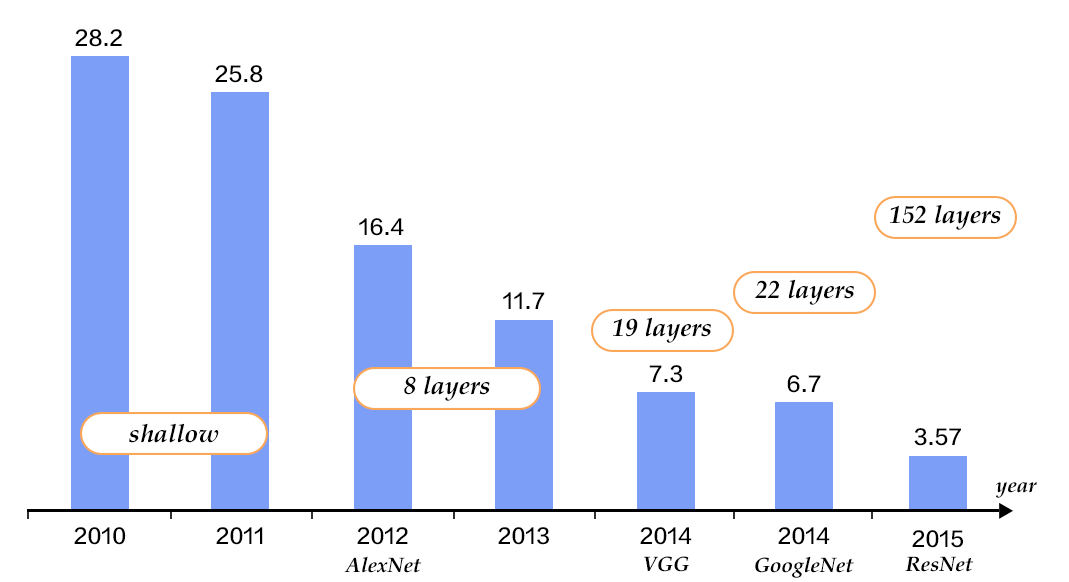### CNN¶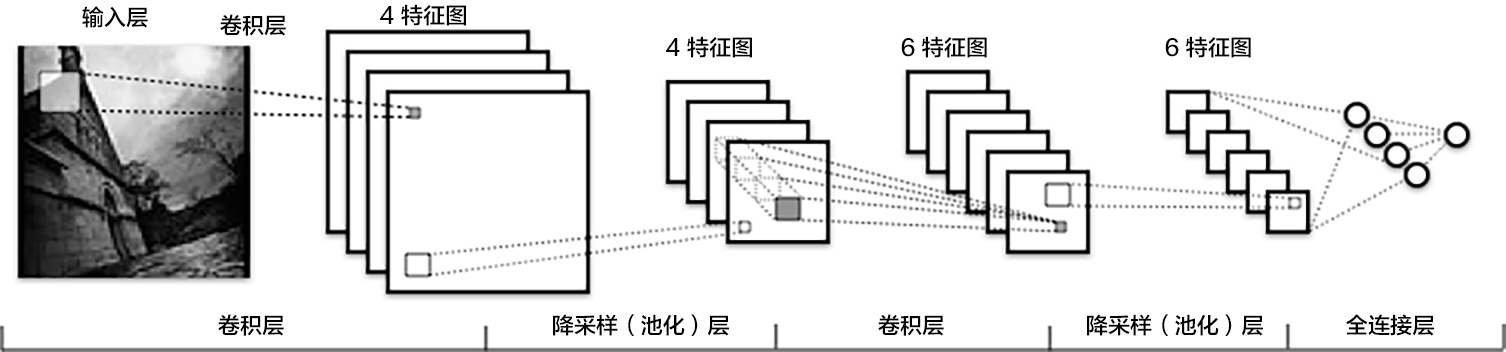• 卷积层(convolution layer): 执行卷积操作提取底层到高层的特征，发掘出图片局部关联性质和空间不变性质。

• 池化层(pooling layer): 执行降采样操作。通过取卷积输出特征图中局部区块的最大值(max-pooling)或者均值(avg-pooling)。降采样也是图像处理中常见的一种操作，可以过滤掉一些不重要的高频信息。

• 全连接层(fully-connected layer，或者fc layer): 输入层到隐藏层的神经元是全部连接的。

• 非线性变化: 卷积层、全连接层后面一般都会接非线性变化函数，例如Sigmoid、Tanh、ReLu等来增强网络的表达能力，在CNN里最常使用的为ReLu激活函数。

• Dropout  : 在模型训练阶段随机让一些隐层节点权重不工作，提高网络的泛化能力，一定程度上防止过拟合。

### VGG¶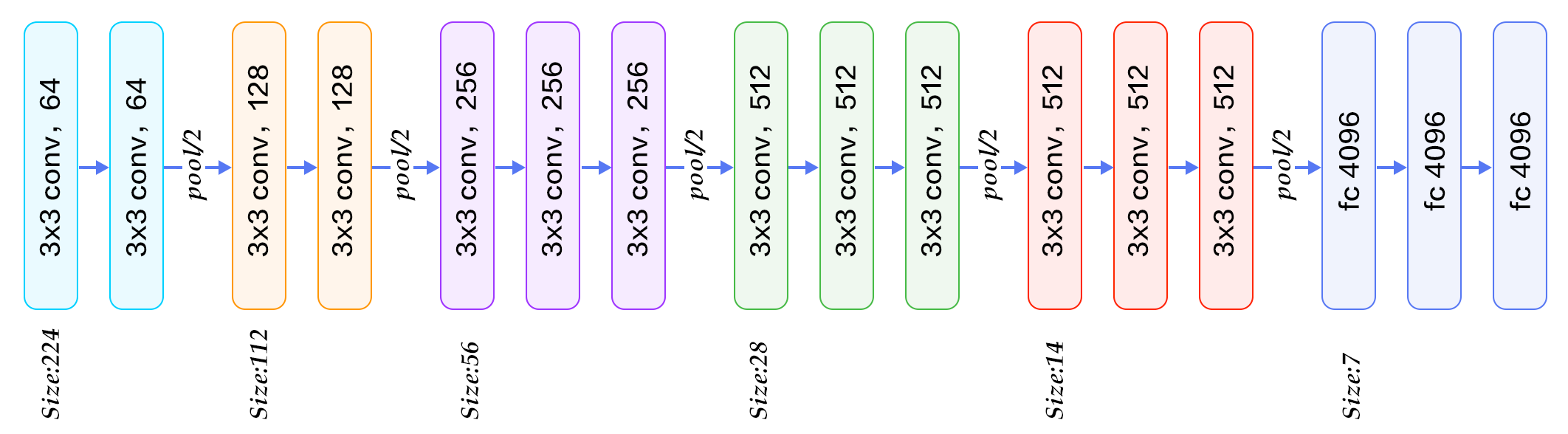NIN模型主要有两个特点：

1. 引入了多层感知卷积网络(Multi-Layer Perceptron Convolution, MLPconv)代替一层线性卷积网络。MLPconv是一个微小的多层卷积网络，即在线性卷积后面增加若干层1x1的卷积，这样可以提取出高度非线性特征。

2. 传统的CNN最后几层一般都是全连接层，参数较多。而NIN模型设计最后一层卷积层包含类别维度大小的特征图，然后采用全局均值池化(Avg-Pooling)替代全连接层，得到类别维度大小的向量，再进行分类。这种替代全连接层的方式有利于减少参数。

Inception模块如下图7所示，图(a)是最简单的设计，输出是3个卷积层和一个池化层的特征拼接。这种设计的缺点是池化层不会改变特征通道数，拼接后会导致特征的通道数较大，经过几层这样的模块堆积后，通道数会越来越大，导致参数和计算量也随之增大。为了改善这个缺点，图(b)引入3个1x1卷积层进行降维，所谓的降维就是减少通道数，同时如NIN模型中提到的1x1卷积也可以修正线性特征。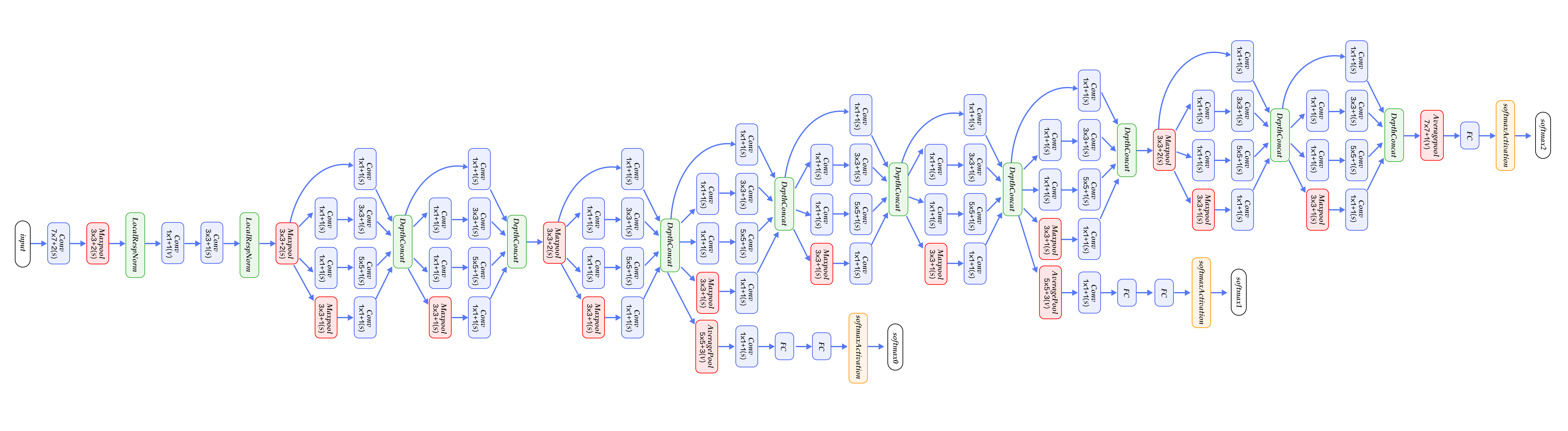### ResNet¶

ResNet(Residual Network)  是2015年ImageNet图像分类、图像物体定位和图像物体检测比赛的冠军。针对随着网络训练加深导致准确度下降的问题，ResNet提出了残差学习方法来减轻训练深层网络的困难。在已有设计思路(BN, 小卷积核，全卷积网络)的基础上，引入了残差模块。每个残差模块包含两条路径，其中一条路径是输入特征的直连通路，另一条路径对该特征做两到三次卷积操作得到该特征的残差，最后再将两条路径上的特征相加。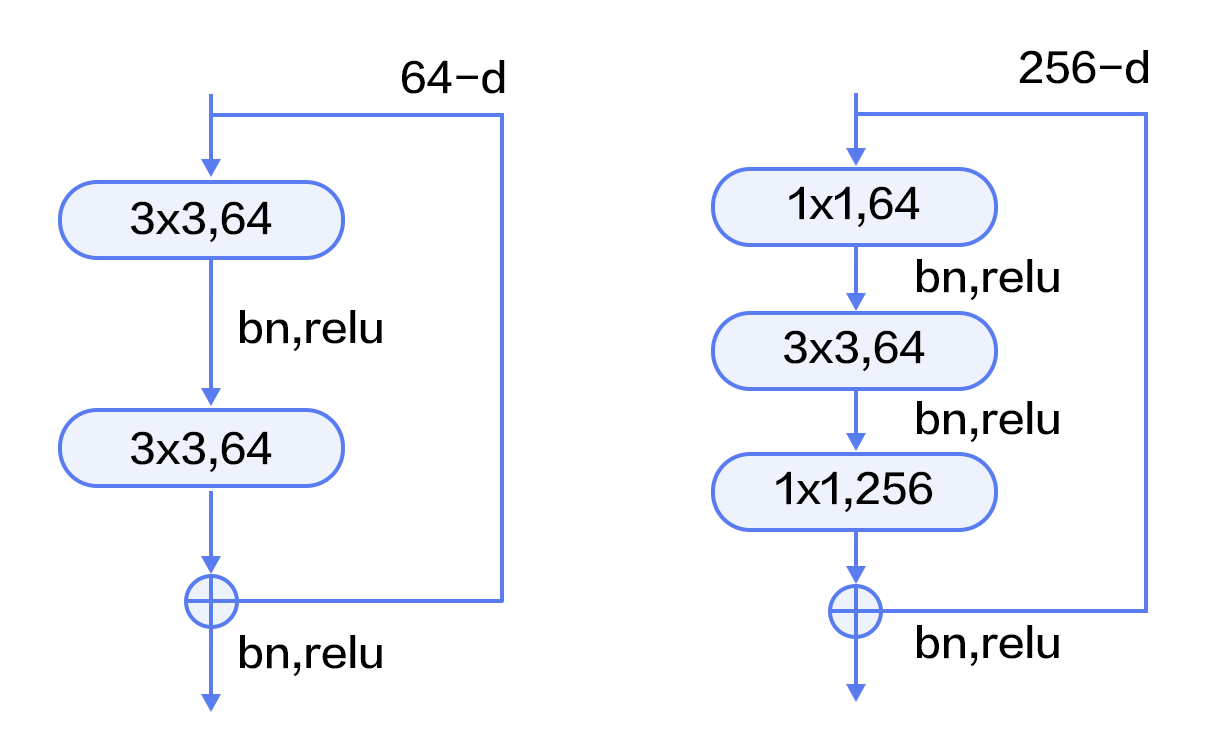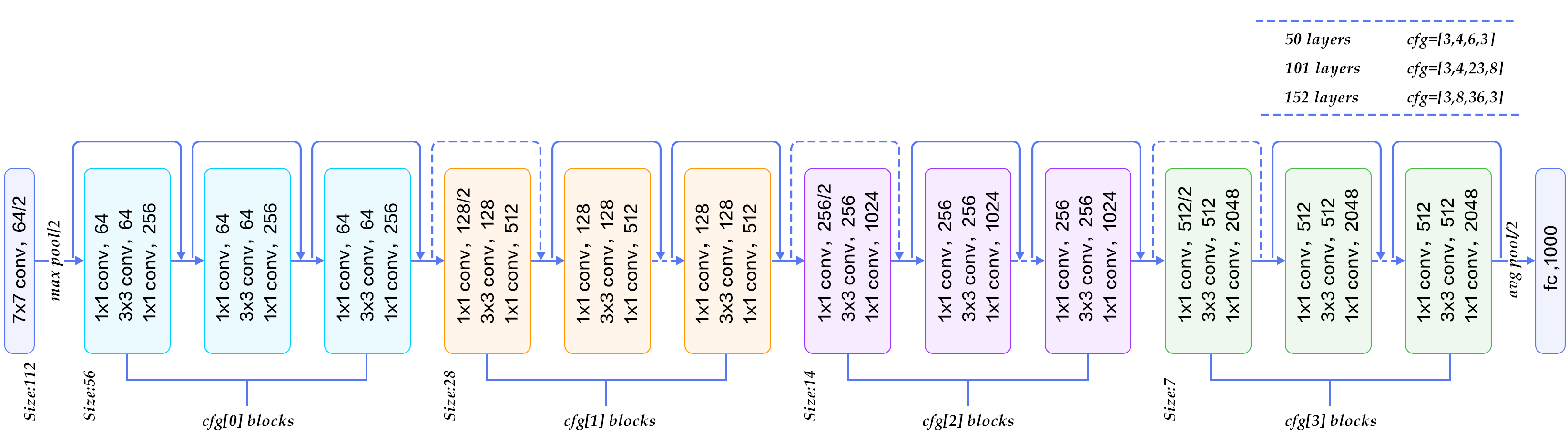## 数据准备¶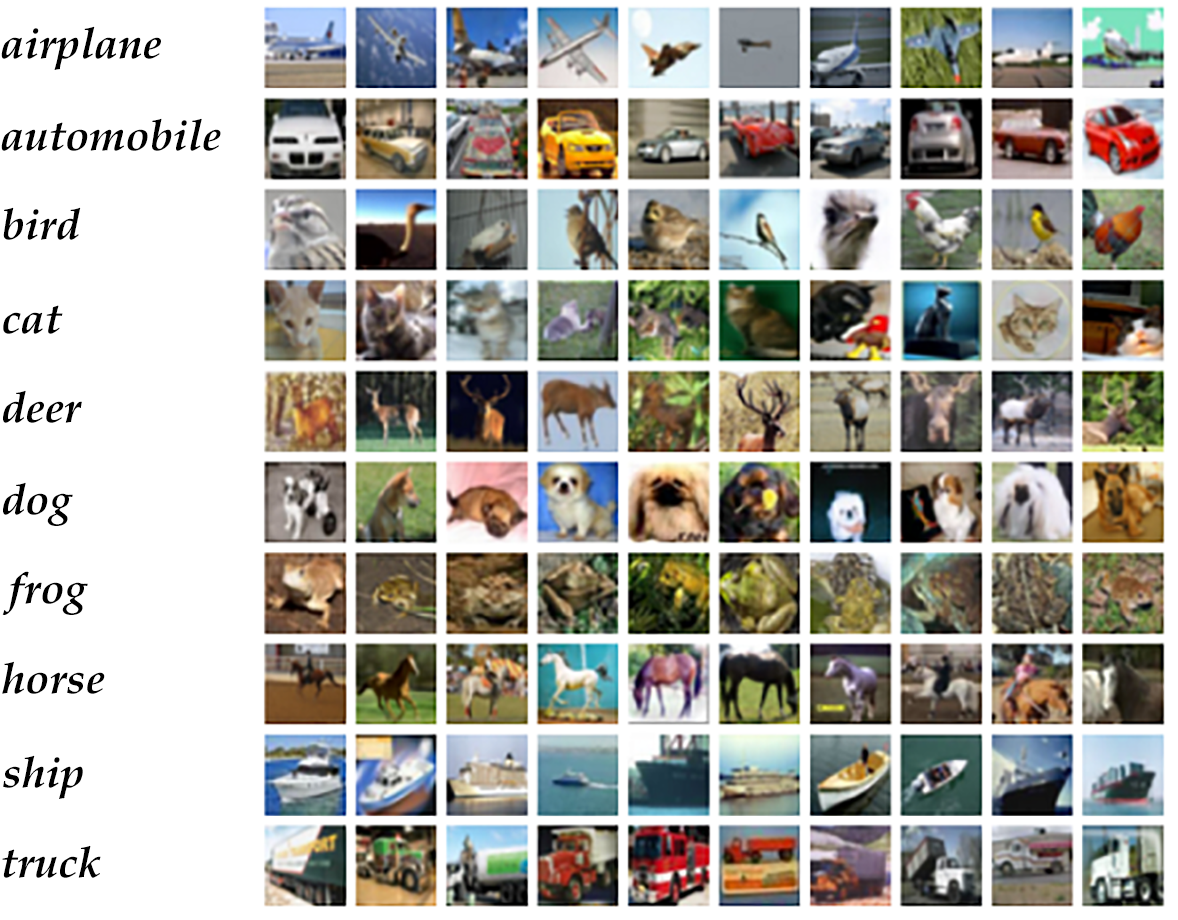Paddle API提供了自动加载cifar数据集模块 `paddle.dataset.cifar`

### 模型结构¶

```
from __future__ import print_function
import numpy
import sys
```

### VGG¶

```def vgg_bn_drop(input):
def conv_block(ipt, num_filter, groups, dropouts):
return fluid.nets.img_conv_group(
input=ipt,
pool_size=2,
pool_stride=2,
conv_num_filter=[num_filter] * groups,
conv_filter_size=3,
conv_act='relu',
conv_with_batchnorm=True,
conv_batchnorm_drop_rate=dropouts,
pool_type='max')

conv1 = conv_block(input, 64, 2, [0.3, 0])
conv2 = conv_block(conv1, 128, 2, [0.4, 0])
conv3 = conv_block(conv2, 256, 3, [0.4, 0.4, 0])
conv4 = conv_block(conv3, 512, 3, [0.4, 0.4, 0])
conv5 = conv_block(conv4, 512, 3, [0.4, 0.4, 0])

drop = fluid.layers.dropout(x=conv5, dropout_prob=0.5)
fc1 = fluid.layers.fc(input=drop, size=512, act=None)
bn = fluid.layers.batch_norm(input=fc1, act='relu')
drop2 = fluid.layers.dropout(x=bn, dropout_prob=0.5)
fc2 = fluid.layers.fc(input=drop2, size=512, act=None)
predict = fluid.layers.fc(input=fc2, size=10, act='softmax')
return predict
```
1. 首先定义了一组卷积网络，即conv_block。卷积核大小为3x3，池化窗口大小为2x2，窗口滑动大小为2，groups决定每组VGG模块是几次连续的卷积操作，dropouts指定Dropout操作的概率。所使用的`img_conv_group`是在`paddle.nets`中预定义的模块，由若干组 Conv->BN->ReLu->Dropout 和 一组 Pooling 组成。

2. 五组卷积操作，即 5个conv_block。 第一、二组采用两次连续的卷积操作。第三、四、五组采用三次连续的卷积操作。每组最后一个卷积后面Dropout概率为0，即不使用Dropout操作。

3. 最后接两层512维的全连接。

4. 在这里，VGG网络首先提取高层特征，随后在全连接层中将其映射到和类别维度大小一致的向量上，最后通过Softmax方法计算图片划为每个类别的概率。

### ResNet¶

ResNet模型的第1、3、4步和VGG模型相同，这里不再介绍。主要介绍第2步即CIFAR10数据集上ResNet核心模块。

• `conv_bn_layer` : 带BN的卷积层。

• `shortcut` : 残差模块的"直连"路径，"直连"实际分两种形式：残差模块输入和输出特征通道数不等时，采用1x1卷积的升维操作；残差模块输入和输出通道相等时，采用直连操作。

• `basicblock` : 一个基础残差模块，即图9左边所示，由两组3x3卷积组成的路径和一条"直连"路径组成。

• `layer_warp` : 一组残差模块，由若干个残差模块堆积而成。每组中第一个残差模块滑动窗口大小与其他可以不同，以用来减少特征图在垂直和水平方向的大小。

```def conv_bn_layer(input,
ch_out,
filter_size,
stride,
act='relu',
bias_attr=False):
tmp = fluid.layers.conv2d(
input=input,
filter_size=filter_size,
num_filters=ch_out,
stride=stride,
act=None,
bias_attr=bias_attr)
return fluid.layers.batch_norm(input=tmp, act=act)

def shortcut(input, ch_in, ch_out, stride):
if ch_in != ch_out:
return conv_bn_layer(input, ch_out, 1, stride, 0, None)
else:
return input

def basicblock(input, ch_in, ch_out, stride):
tmp = conv_bn_layer(input, ch_out, 3, stride, 1)
tmp = conv_bn_layer(tmp, ch_out, 3, 1, 1, act=None, bias_attr=True)
short = shortcut(input, ch_in, ch_out, stride)

def layer_warp(block_func, input, ch_in, ch_out, count, stride):
tmp = block_func(input, ch_in, ch_out, stride)
for i in range(1, count):
tmp = block_func(tmp, ch_out, ch_out, 1)
return tmp
```

`resnet_cifar10` 的连接结构主要有以下几个过程。

1. 底层输入连接一层 `conv_bn_layer`，即带BN的卷积层。

2. 然后连接3组残差模块即下面配置3组 `layer_warp` ，每组采用图 10 左边残差模块组成。

3. 最后对网络做均值池化并返回该层。

```def resnet_cifar10(ipt, depth=32):
# depth should be one of 20, 32, 44, 56, 110, 1202
assert (depth - 2) % 6 == 0
n = (depth - 2) // 6
nStages = {16, 64, 128}
conv1 = conv_bn_layer(ipt, ch_out=16, filter_size=3, stride=1, padding=1)
res1 = layer_warp(basicblock, conv1, 16, 16, n, 1)
res2 = layer_warp(basicblock, res1, 16, 32, n, 2)
res3 = layer_warp(basicblock, res2, 32, 64, n, 2)
pool = fluid.layers.pool2d(
input=res3, pool_size=8, pool_type='avg', pool_stride=1)
predict = fluid.layers.fc(input=pool, size=10, act='softmax')
return predict
```

## Inference Program 配置¶

```def inference_program():
# The image is 32 * 32 with RGB representation.
data_shape = [None, 3, 32, 32]
images = fluid.data(name='pixel', shape=data_shape, dtype='float32')

predict = resnet_cifar10(images, 32)
# predict = vgg_bn_drop(images) # un-comment to use vgg net
return predict
```

## Train Program 配置¶

```def train_program():
predict = inference_program()

label = fluid.data(name='label', shape=[None,1], dtype='int64')
cost = fluid.layers.cross_entropy(input=predict, label=label)
avg_cost = fluid.layers.mean(cost)
accuracy = fluid.layers.accuracy(input=predict, label=label)
return [avg_cost, accuracy, predict]
```

## Optimizer Function 配置¶

```def optimizer_program():
```

## 训练模型¶

### Data Feeders 配置¶

`cifar.train10()` 每次产生一条样本，在完成shuffle和batch之后，作为训练的输入。

```# Each batch will yield 128 images
BATCH_SIZE = 128

batch_size=BATCH_SIZE)

# Reader for testing. A separated data set for testing.
```

### Trainer 程序的实现¶

```use_cuda = False
place = fluid.CUDAPlace(0) if use_cuda else fluid.CPUPlace()

feed_order = ['pixel', 'label']

main_program = fluid.default_main_program()
star_program = fluid.default_startup_program()

avg_cost, acc, predict = train_program()

# Test program
test_program = main_program.clone(for_test=True)

optimizer = optimizer_program()
optimizer.minimize(avg_cost)

exe = fluid.Executor(place)

EPOCH_NUM = 2

# For training test cost
count = 0
feed_var_list = [
program.global_block().var(var_name) for var_name in feed_order
]
feeder_test = fluid.DataFeeder(
feed_list=feed_var_list, place=place)
test_exe = fluid.Executor(place)
accumulated = len([avg_cost, acc]) * 
avg_cost_np = test_exe.run(program=program,
feed=feeder_test.feed(test_data),
fetch_list=[avg_cost, acc])
accumulated = [x + x for x in zip(accumulated, avg_cost_np)]
count += 1
return [x / count for x in accumulated]
```

### 训练主循环以及过程输出¶

```params_dirname = "image_classification_resnet.inference.model"

train_prompt = "Train cost"
test_prompt = "Test cost"
plot_cost = Ploter(test_prompt,train_prompt)

# main train loop.
def train_loop():
feed_var_list_loop = [
main_program.global_block().var(var_name) for var_name in feed_order
]
feeder = fluid.DataFeeder(
feed_list=feed_var_list_loop, place=place)
exe.run(star_program)

step = 0
for pass_id in range(EPOCH_NUM):
avg_loss_value = exe.run(main_program,
feed=feeder.feed(data_train),
fetch_list=[avg_cost, acc])
if step % 1 == 0:
plot_cost.append(train_prompt, step, avg_loss_value)
plot_cost.plot()
step += 1

avg_cost_test, accuracy_test = train_test(test_program,
plot_cost.append(test_prompt, step, avg_cost_test)

# save parameters
if params_dirname is not None:
fluid.io.save_inference_model(params_dirname, ["pixel"],
[predict], exe)
```

### 训练¶

```train_loop()
```

```Pass 0, Batch 0, Cost 3.869598, Acc 0.164062
...................................................................................................
Pass 100, Batch 0, Cost 1.481038, Acc 0.460938
...................................................................................................
Pass 200, Batch 0, Cost 1.340323, Acc 0.523438
...................................................................................................
Pass 300, Batch 0, Cost 1.223424, Acc 0.593750
..........................................................................................
Test with Pass 0, Loss 1.1, Acc 0.6
```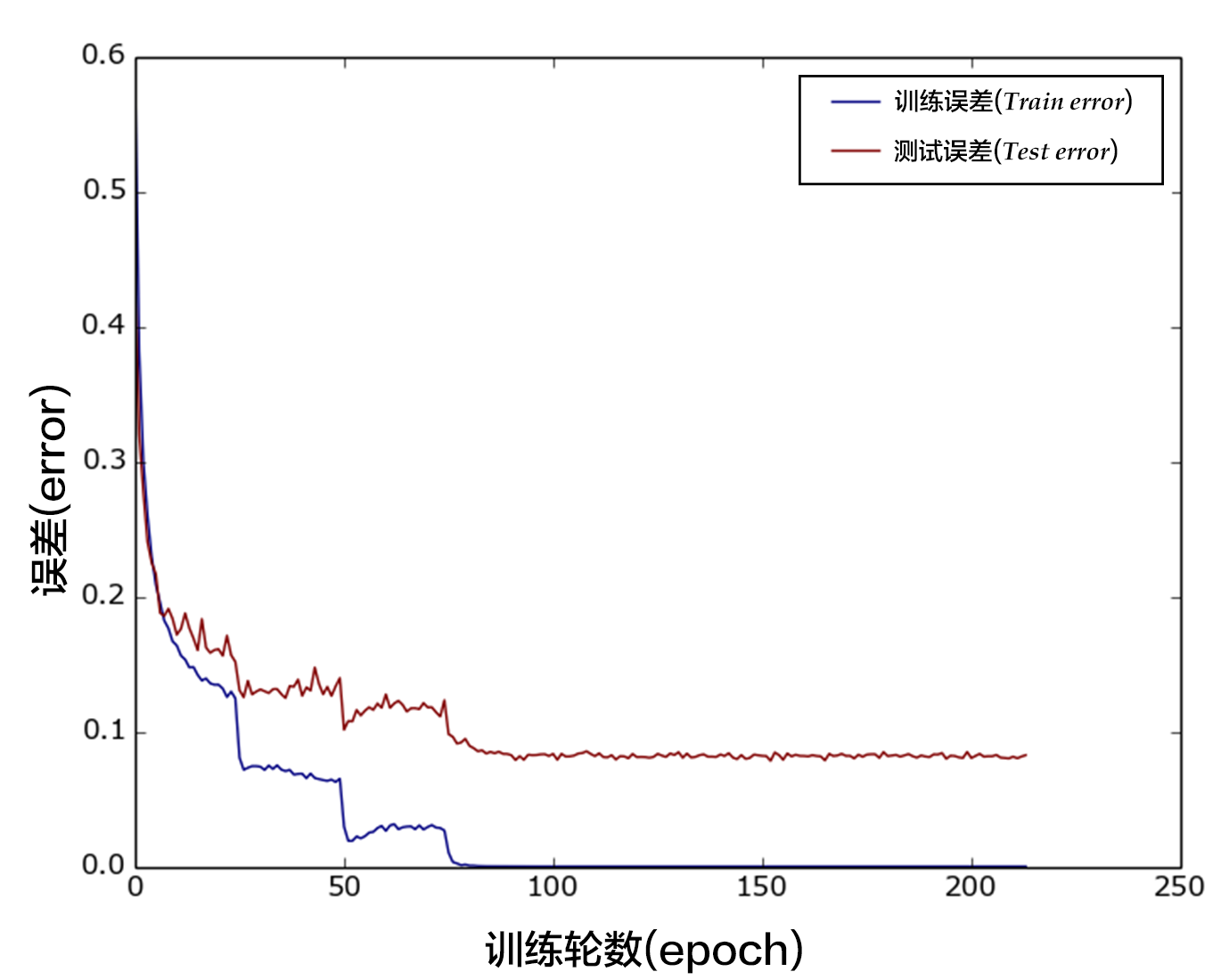## 应用模型¶

### 生成预测输入数据¶

`dog.png` 是一张小狗的图片. 我们将它转换成 `numpy` 数组以满足`feeder`的格式.

```# Prepare testing data.
from PIL import Image
import os

im = Image.open(file)
im = im.resize((32, 32), Image.ANTIALIAS)

im = numpy.array(im).astype(numpy.float32)
# The storage order of the loaded image is W(width),
# the CHW order, so transpose them.
im = im.transpose((2, 0, 1))  # CHW
im = im / 255.0

# Add one dimension to mimic the list format.
im = numpy.expand_dims(im, axis=0)
return im

cur_dir = os.getcwd()
```

### Inferencer 配置和预测¶

```place = fluid.CUDAPlace(0) if use_cuda else fluid.CPUPlace()
exe = fluid.Executor(place)
inference_scope = fluid.core.Scope()

with fluid.scope_guard(inference_scope):

[inference_program, feed_target_names,

# Construct feed as a dictionary of {feed_target_name: feed_target_data}
# and results will contain a list of data corresponding to fetch_targets.
results = exe.run(inference_program,
feed={feed_target_names: img},
fetch_list=fetch_targets)

# infer label
label_list = [
"airplane", "automobile", "bird", "cat", "deer", "dog", "frog", "horse",
"ship", "truck"
]

print("infer results: %s" % label_list[numpy.argmax(results)])
```

## 参考文献¶

 D. G. Lowe, Distinctive image features from scale-invariant keypoints. IJCV, 60(2):91-110, 2004.

 N. Dalal, B. Triggs, Histograms of Oriented Gradients for Human Detection, Proc. IEEE Conf. Computer Vision and Pattern Recognition, 2005.

 Ahonen, T., Hadid, A., and Pietikinen, M. (2006). Face description with local binary patterns: Application to face recognition. PAMI, 28.

 J. Sivic, A. Zisserman, Video Google: A Text Retrieval Approach to Object Matching in Videos, Proc. Ninth Int'l Conf. Computer Vision, pp. 1470-1478, 2003.

 B. Olshausen, D. Field, Sparse Coding with an Overcomplete Basis Set: A Strategy Employed by V1?, Vision Research, vol. 37, pp. 3311-3325, 1997.

 Wang, J., Yang, J., Yu, K., Lv, F., Huang, T., and Gong, Y. (2010). Locality-constrained Linear Coding for image classification. In CVPR.

 Perronnin, F., Sánchez, J., & Mensink, T. (2010). Improving the fisher kernel for large-scale image classification. In ECCV (4).

 Lin, Y., Lv, F., Cao, L., Zhu, S., Yang, M., Cour, T., Yu, K., and Huang, T. (2011). Large-scale image clas- sification: Fast feature extraction and SVM training. In CVPR.

 Krizhevsky, A., Sutskever, I., and Hinton, G. (2012). ImageNet classification with deep convolutional neu- ral networks. In NIPS.

 G.E. Hinton, N. Srivastava, A. Krizhevsky, I. Sutskever, and R.R. Salakhutdinov. Improving neural networks by preventing co-adaptation of feature detectors. arXiv preprint arXiv:1207.0580, 2012.

 K. Chatfield, K. Simonyan, A. Vedaldi, A. Zisserman. Return of the Devil in the Details: Delving Deep into Convolutional Nets. BMVC, 2014。

 Szegedy, C., Liu, W., Jia, Y., Sermanet, P., Reed, S., Anguelov, D., Erhan, D., Vanhoucke, V., Rabinovich, A., Going deeper with convolutions. In: CVPR. (2015)

 Lin, M., Chen, Q., and Yan, S. Network in network. In Proc. ICLR, 2014.

 S. Ioffe and C. Szegedy. Batch normalization: Accelerating deep network training by reducing internal covariate shift. In ICML, 2015.

 K. He, X. Zhang, S. Ren, J. Sun. Deep Residual Learning for Image Recognition. CVPR 2016.

 Szegedy, C., Vanhoucke, V., Ioffe, S., Shlens, J., Wojna, Z. Rethinking the incep-tion architecture for computer vision. In: CVPR. (2016).

 Szegedy, C., Ioffe, S., Vanhoucke, V. Inception-v4, inception-resnet and the impact of residual connections on learning. arXiv:1602.07261 (2016).

 Everingham, M., Eslami, S. M. A., Van Gool, L., Williams, C. K. I., Winn, J. and Zisserman, A. The Pascal Visual Object Classes Challenge: A Retrospective. International Journal of Computer Vision, 111(1), 98-136, 2015.

 He, K., Zhang, X., Ren, S., and Sun, J. Delving Deep into Rectifiers: Surpassing Human-Level Performance on ImageNet Classification. ArXiv e-prints, February 2015.

 http://deeplearning.net/tutorial/lenet.html

 https://www.cs.toronto.edu/~kriz/cifar.html

 http://cs231n.github.io/classification/